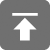# 工学1号馆

home

## C语言中do…while(0)技巧

Wu Yudong    June 27, 2015     C   456

### 1、避免goto语句：

#defien N 10

bool Execute()
{
// 分配资源
int *p = (int *)malloc(N * sizeof(int));
bool bOk = true;

// 执行并进行错误处理
bOk = func1();
if(!bOk)
{
free(p);
p = NULL;
return false;
}

bOk = func2();
if(!bOk)
{
free(p);
p = NULL;
return false;
}

bOk = func3();
if(!bOk)
{
free(p);
p = NULL;
return false;
}

// ..........

// 执行成功，释放资源并返回
free(p);
p = NULL;
return true;
}

#defien N 10

bool Execute()
{
// 分配资源
int *p = (int *)malloc(N * sizeof(int));
bool bOk = true;

// 执行并进行错误处理
bOk = func1();
if(!bOk) goto errorhandle;

bOk = func2();
if(!bOk) goto errorhandle;

bOk = func3();
if(!bOk) goto errorhandle;

// ..........

// 执行成功，释放资源并返回
free(p);
p = NULL;
return true;

errorhandle:
free(p);
p = NULL;
return false;
}

#defien N 10

bool Execute()
{
//分配资源
int *p = (int *)malloc(N * sizeof(int));
bool bOK = true;

do {
//执行并进行错误处理
bOK = fun1();
if(!bOK) break;

bOK = fun2();
if(!bOK) break;

bOK = fun3();
if(!bOK) break;

//.........
}  while(0);

//释放资源

free(p);
p = NULL;
return bOK;
}

### 2、避免空声明在编译时出现警告：

#define FOO do { } while(0)

### 3、编写符合习惯的代码块：

#define exch(x,y) { int tmp; tmp=x; x=y; y=tmp; }

if (x > y)
exch(x,y);          // 分支 1
else
do_something();     // 分支 2

if (x > y) {
int tmp;
tmp = x;
x = y;
y = tmp;
}
;                           // 空语句
else                        // ERROR!!!
do_something();

if (x > y)
do {
int tmp;
tmp = x;
x = y;
y = tmp;
} while(0);
else
do_something();

#define exch(x,y)       do {\
int tmp;\
tmp = x;\
x = y;\
y = tmp;\
} while(0)

### 4、在条件语句中使用复杂的宏：

#define FOO(x) \
printf("arg is %s\n", x); \
do_something_useful(x);

if (blah == 2)
FOO(blah);

if (blah == 2)
printf("arg is %s\n", blah);
do_something_useful(blah);;

if (blah == 2)
do {
printf("arg is %s\n", blah);
do_something_useful(blah);
} while (0);

#define  FOO(x) do { \
printf("arg is %s\n", blah);\
do_something_useful(blah);\
} while (0)